# 1、最长有效括号

输入: "(()"



输入: ")()())"



## 1.1、解题思路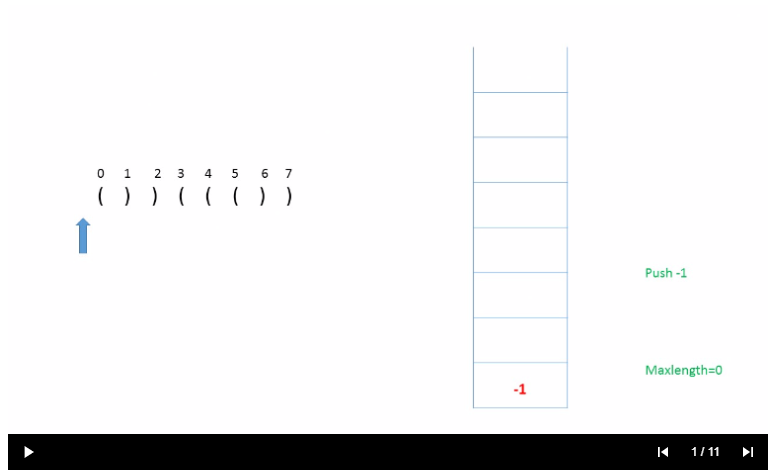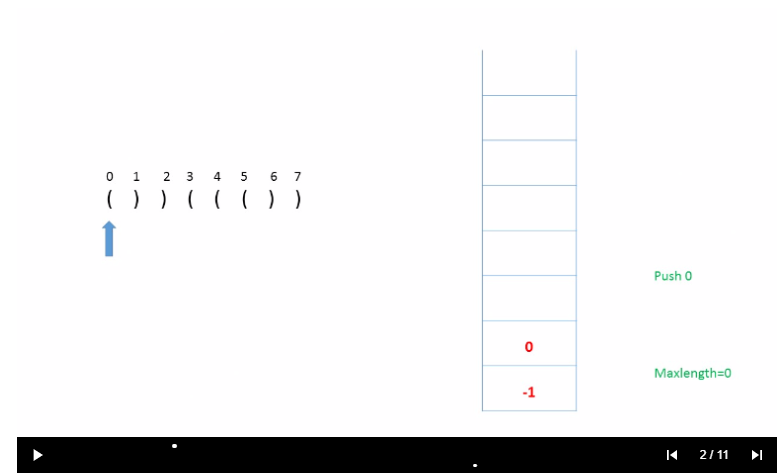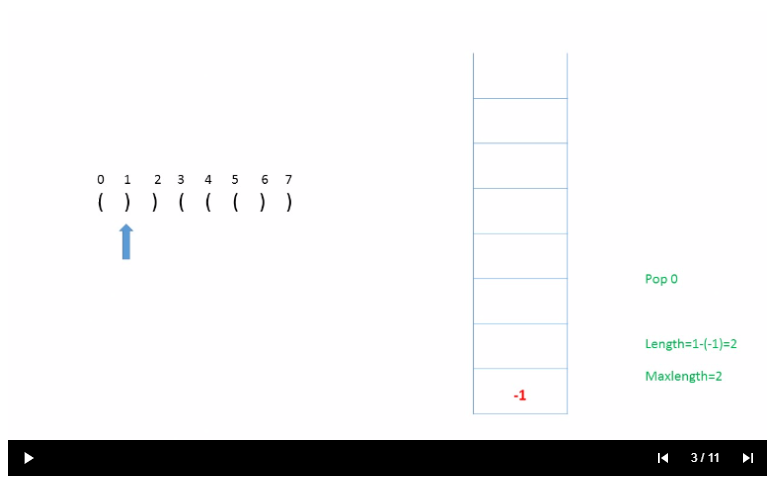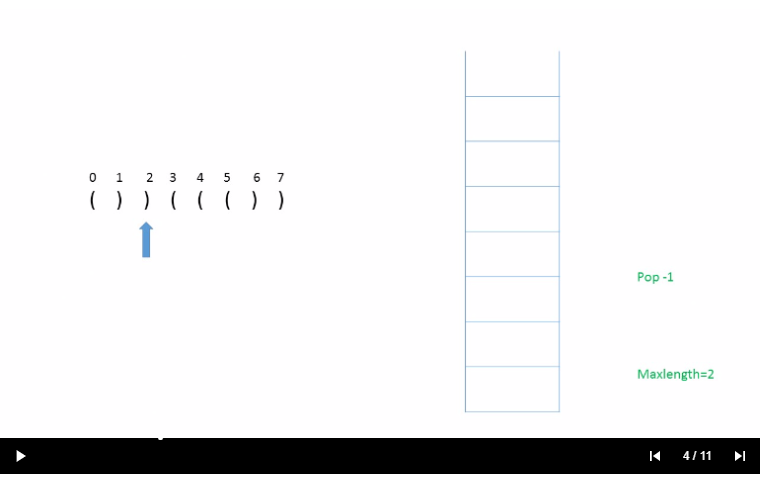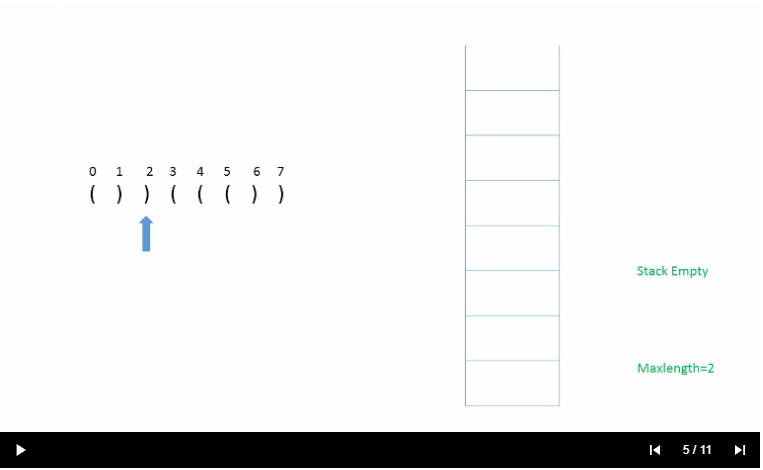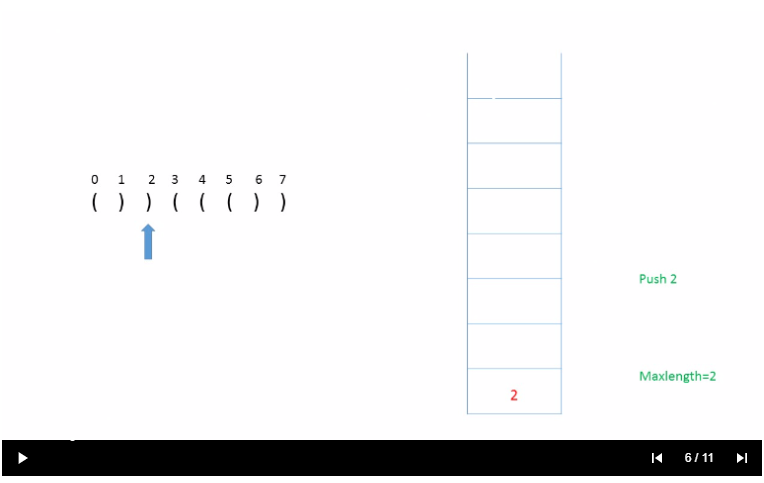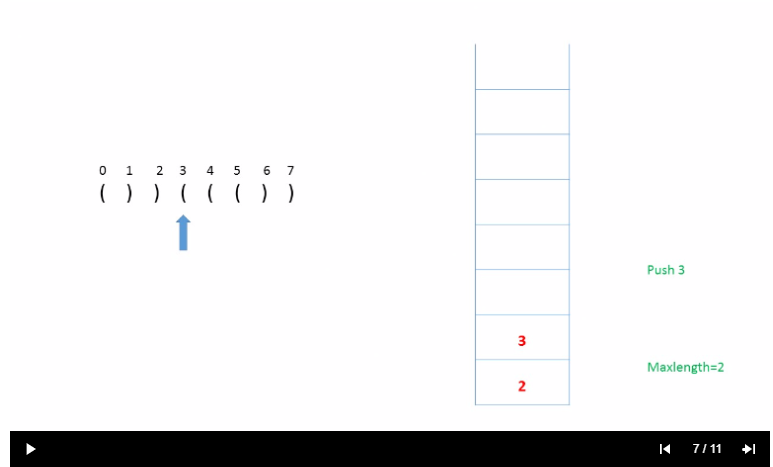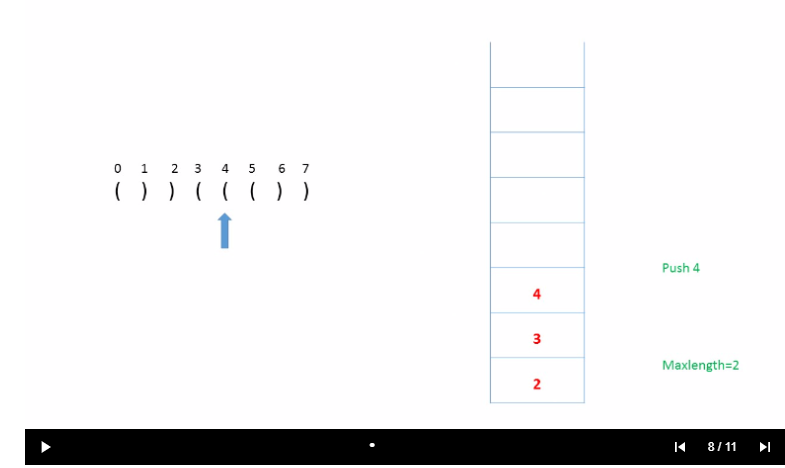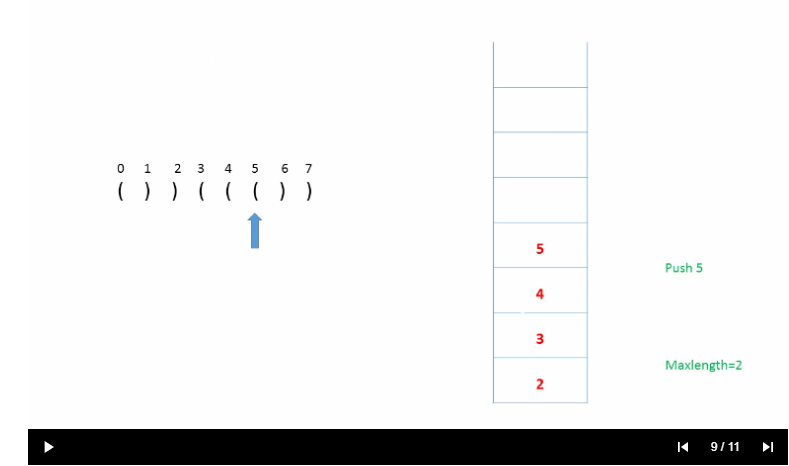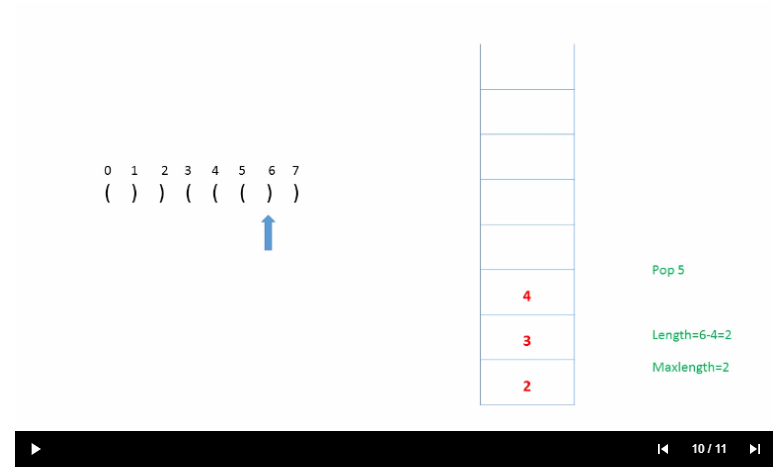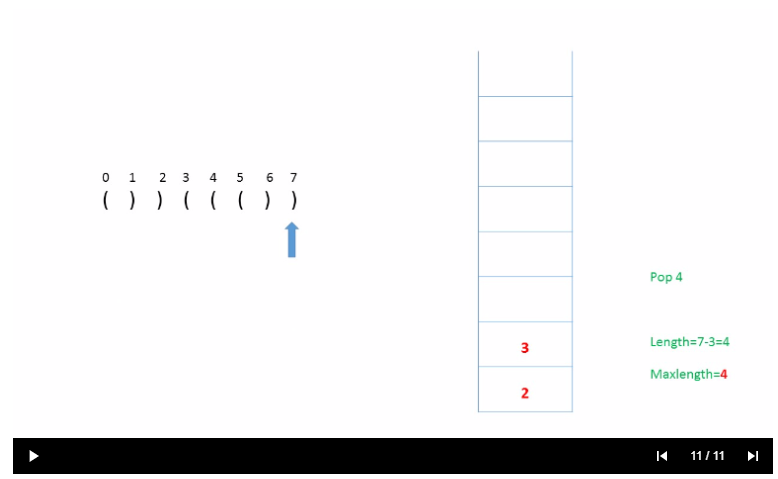## 1.2、算法

    public int longestValidParentheses(String s) {
int max = 0;
Stack<Integer> stack = new Stack<>();
//占位置
stack.push(-1);
for (int i = 0; i < s.length(); i++) {
//如果是 ( 放心入栈
if (s.charAt(i) == '(') {
stack.push(i);
} else {
//正常情况下，出栈的是( ，但是这个时候栈里面是 -1 或者是 ) 那么取出来栈就变成空了，所以如果是空的情况，还会把它放进去
stack.pop();
if (stack.empty()) {
//不能让栈变成空，因为有值的情况才能进行截取
stack.push(i);
} else {
//截取长度
max = Math.max(max, i - stack.peek());
}
}
}
return max;
}


## 1.3、测试

  @Test
public void test() {
System.out.println(longestValidParentheses("()"));
}

1